# Adaptive MPC Control of Nonlinear Chemical Reactor Using Linear Parameter-Varying System

This example shows how to use an adaptive MPC controller to control a nonlinear continuous stirred tank reactor (CSTR) as it transitions from low conversion rate to high conversion rate.

A linear parameter varying (LPV) system consisting of three linear plant models is constructed offline to describe the local plant dynamics across the operating range. The adaptive MPC controller then uses the LPV system to update the internal predictive model at each control interval and achieves nonlinear control successfully.

### About the Continuous Stirred Tank Reactor

A continuously stirred tank reactor (CSTR) is a common chemical system in the process industry. A schematic of the CSTR system is: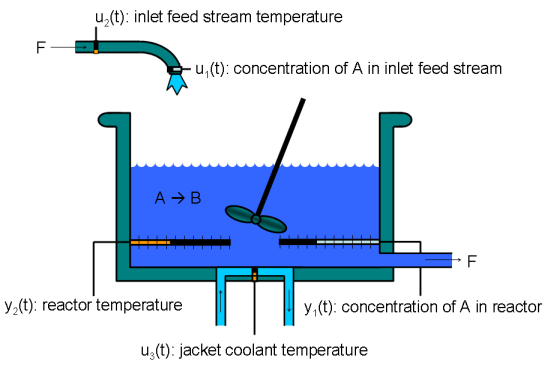This system is a jacketed non-adiabatic tank reactor described extensively in . The vessel is assumed to be perfectly mixed, and a single first-order exothermic and irreversible reaction, A --> B, takes place. The inlet stream of reagent A is fed to the tank at a constant volumetric rate. The product stream exits continuously at the same volumetric rate, and liquid density is constant. Thus, the volume of reacting liquid is constant.

The inputs of the CSTR model are: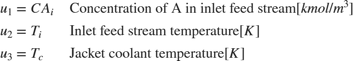The outputs of the model, which are also the model states, are: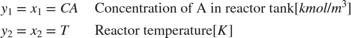The control objective is to maintain the concentration of reagent A,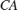at its desired setpoint, which changes over time when the reactor transitions from a low conversion rate to a high conversion rate. The coolant temperature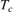is the manipulated variable used by the MPC controller to track the reference. The inlet feed stream concentration and temperature are assumed to be constant. The Simulink model mpc_cstr_plant implements the nonlinear CSTR plant. For more information on the CSTR reactor and related examples, see CSTR Model.

It is well known that the CSTR dynamics are strongly nonlinear with respect to reactor temperature variations and can be open-loop unstable during the transition from one operating condition to another. A single MPC controller designed at a particular operating condition cannot give satisfactory control performance over a wide operating range.

To control the nonlinear CSTR plant with linear MPC control technique, you have a few options:

• If a linear plant model cannot be obtained at run time, first you need to obtain several linear plant models offline at different operating conditions that cover the typical operating range. Next, you can choose one of the two approaches to implement the MPC control strategy:

(1) Design several MPC controllers offline, one for each plant model. At run time, use the Multiple MPC Controller block, which switches between controllers based on a desired scheduling strategy. For more details, see Gain-Scheduled MPC Control of Nonlinear Chemical Reactor. Use this approach when the plant models have different orders or time delays.

(2) Design one MPC controller offline at the initial operating point. At run time, use Adaptive MPC Controller block (updating predictive model at each control interval) together with Linear Parameter Varying (LPV) System block (supplying linear plant model with a scheduling strategy) as shown in this example. Use this approach when all the plant models have the same order and time delay.

• If a linear plant model can be obtained at run time, you should use the Adaptive MPC Controller block to achieve nonlinear control. There are two typical ways to obtain a linear plant model online:

(1) Use successive linearization. For more details, see Adaptive MPC Control of Nonlinear Chemical Reactor Using Successive Linearization. Use this approach when a nonlinear plant model is available and can be linearized at run time.

(2) Use online estimation to identify a linear model when loop is closed. For more details, see Adaptive MPC Control of Nonlinear Chemical Reactor Using Online Model Estimation. Use this approach when a linear plant model cannot be obtained from either an LPV system or successive linearization.

### Obtain Linear Plant Model at Initial Operating Condition

First, obtain a linear plant model at the initial operating condition, where CAi is 10 kmol/m^3, and both Ti and Tc are 298.15 K. To generate the linear state-space system from the Simulink model, use functions such as operspec, findop, and linearize from Simulink Control Design.

Create operating point specification.

plant_mdl = 'mpc_cstr_plant'; op = operspec(plant_mdl); 

Specify the known feed concentration at the initial condition.

op.Inputs(1).u = 10; op.Inputs(1).Known = true; 

Specify the known feed temperature at the initial condition.

op.Inputs(2).u = 298.15; op.Inputs(2).Known = true; 

Specify the known coolant temperature at the initial condition.

op.Inputs(3).u = 298.15; op.Inputs(3).Known = true; 

Compute the initial condition.

[op_point,op_report] = findop(plant_mdl,op); 
 Operating point search report: --------------------------------- opreport = Operating point search report for the Model mpc_cstr_plant. (Time-Varying Components Evaluated at time t=0) Operating point specifications were successfully met. States: ---------- Min x Max dxMin dx dxMax ___________ ___________ ___________ ___________ ___________ ___________ (1.) mpc_cstr_plant/CSTR/Integrator 0 311.2639 Inf 0 8.1176e-11 0 (2.) mpc_cstr_plant/CSTR/Integrator1 0 8.5698 Inf 0 -6.8709e-12 0 Inputs: ---------- Min u Max ______ ______ ______ (1.) mpc_cstr_plant/CAi 10 10 10 (2.) mpc_cstr_plant/Ti 298.15 298.15 298.15 (3.) mpc_cstr_plant/Tc 298.15 298.15 298.15 Outputs: ---------- Min y Max ________ ________ ________ (1.) mpc_cstr_plant/T -Inf 311.2639 Inf (2.) mpc_cstr_plant/CA -Inf 8.5698 Inf 

Obtain nominal values of x, y, and u.

x0_initial = [op_report.States(1).x; op_report.States(2).x]; y0_initial = [op_report.Outputs(1).y; op_report.Outputs(2).y]; u0_initial = [op_report.Inputs(1).u; op_report.Inputs(2).u; op_report.Inputs(3).u]; 

Obtain a linear model at the initial condition.

plant_initial = linearize(plant_mdl,op_point); 

Discretize the plant model.

Ts = 0.5; plant_initial = c2d(plant_initial,Ts); 

Specify signal types and names used in MPC.

plant_initial.InputGroup.UnmeasuredDisturbances = [1 2]; plant_initial.InputGroup.ManipulatedVariables = 3; plant_initial.OutputGroup.Measured = [1 2]; plant_initial.InputName = {'CAi','Ti','Tc'}; plant_initial.OutputName = {'T','CA'}; 

### Obtain Linear Plant Model at Intermediate Operating Condition

Create the operating point specification.

op = operspec(plant_mdl); 

Specify the feed concentration.

op.Inputs(1).u = 10; op.Inputs(1).Known = true; 

Specify the feed temperature.

op.Inputs(2).u = 298.15; op.Inputs(2).Known = true; 

Specify the reactor concentration.

op.Outputs(2).y = 5.5; op.Outputs(2).Known = true; 

[op_point,op_report] = findop(plant_mdl,op); 
 Operating point search report: --------------------------------- opreport = Operating point search report for the Model mpc_cstr_plant. (Time-Varying Components Evaluated at time t=0) Operating point specifications were successfully met. States: ---------- Min x Max dxMin dx dxMax ___________ ___________ ___________ ___________ ___________ ___________ (1.) mpc_cstr_plant/CSTR/Integrator 0 339.4282 Inf 0 3.4187e-08 0 (2.) mpc_cstr_plant/CSTR/Integrator1 0 5.5 Inf 0 -2.8686e-09 0 Inputs: ---------- Min u Max _______ _______ _______ (1.) mpc_cstr_plant/CAi 10 10 10 (2.) mpc_cstr_plant/Ti 298.15 298.15 298.15 (3.) mpc_cstr_plant/Tc -Inf 298.222 Inf Outputs: ---------- Min y Max ________ ________ ________ (1.) mpc_cstr_plant/T -Inf 339.4282 Inf (2.) mpc_cstr_plant/CA 5.5 5.5 5.5 

Obtain nominal values of x, y, and u.

x0_intermediate = [op_report.States(1).x; op_report.States(2).x]; y0_intermediate = [op_report.Outputs(1).y; op_report.Outputs(2).y]; u0_intermediate = [op_report.Inputs(1).u; op_report.Inputs(2).u; op_report.Inputs(3).u]; 

Obtain a linear model at the initial condition.

plant_intermediate = linearize(plant_mdl,op_point); 

Discretize the plant model

plant_intermediate = c2d(plant_intermediate,Ts); 

Specify signal types and names used in MPC.

plant_intermediate.InputGroup.UnmeasuredDisturbances = [1 2]; plant_intermediate.InputGroup.ManipulatedVariables = 3; plant_intermediate.OutputGroup.Measured = [1 2]; plant_intermediate.InputName = {'CAi','Ti','Tc'}; plant_intermediate.OutputName = {'T','CA'}; 

### Obtain Linear Plant Model at Final Operating Condition

Create the operating point specification.

op = operspec(plant_mdl); 

Specify the feed concentration.

op.Inputs(1).u = 10; op.Inputs(1).Known = true; 

Specify the feed temperature.

op.Inputs(2).u = 298.15; op.Inputs(2).Known = true; 

Specify the reactor concentration.

op.Outputs(2).y = 2; op.Outputs(2).Known = true; 

[op_point,op_report] = findop(plant_mdl,op); 
 Operating point search report: --------------------------------- opreport = Operating point search report for the Model mpc_cstr_plant. (Time-Varying Components Evaluated at time t=0) Operating point specifications were successfully met. States: ---------- Min x Max dxMin dx dxMax ___________ ___________ ___________ ___________ ___________ ___________ (1.) mpc_cstr_plant/CSTR/Integrator 0 373.1311 Inf 0 5.7298e-11 0 (2.) mpc_cstr_plant/CSTR/Integrator1 0 2 Inf 0 -4.7411e-12 0 Inputs: ---------- Min u Max ________ ________ ________ (1.) mpc_cstr_plant/CAi 10 10 10 (2.) mpc_cstr_plant/Ti 298.15 298.15 298.15 (3.) mpc_cstr_plant/Tc -Inf 305.2015 Inf Outputs: ---------- Min y Max ________ ________ ________ (1.) mpc_cstr_plant/T -Inf 373.1311 Inf (2.) mpc_cstr_plant/CA 2 2 2 

Obtain nominal values of x, y, and u.

x0_final = [op_report.States(1).x; op_report.States(2).x]; y0_final = [op_report.Outputs(1).y; op_report.Outputs(2).y]; u0_final = [op_report.Inputs(1).u; op_report.Inputs(2).u; op_report.Inputs(3).u]; 

Obtain a linear model at the initial condition.

plant_final = linearize(plant_mdl,op_point); 

Discretize the plant model

plant_final = c2d(plant_final,Ts); 

Specify signal types and names used in MPC.

plant_final.InputGroup.UnmeasuredDisturbances = [1 2]; plant_final.InputGroup.ManipulatedVariables = 3; plant_final.OutputGroup.Measured = [1 2]; plant_final.InputName = {'CAi','Ti','Tc'}; plant_final.OutputName = {'T','CA'}; 

### Construct Linear Parameter-Varying System

Use an LTI array to store the three linear plant models.

lpv(:,:,1) = plant_initial; lpv(:,:,2) = plant_intermediate; lpv(:,:,3) = plant_final; 

Specify reactor temperature T as the scheduling parameter.

lpv.SamplingGrid = struct( ... 'T',[y0_initial(1); y0_intermediate(1); y0_final(1)]); 

Specify nominal values for plant inputs, outputs, and states at each steady-state operating point.

lpv_u0(:,:,1) = u0_initial; lpv_u0(:,:,2) = u0_intermediate; lpv_u0(:,:,3) = u0_final; lpv_y0(:,:,1) = y0_initial; lpv_y0(:,:,2) = y0_intermediate; lpv_y0(:,:,3) = y0_final; lpv_x0(:,:,1) = x0_initial; lpv_x0(:,:,2) = x0_intermediate; lpv_x0(:,:,3) = x0_final; 

You do not need to provide input signal u to the LPV System block because plant output signal y is not used in this example.

### Design MPC Controller at Initial Operating Condition

You design an MPC controller at the initial operating condition. The controller settings such as horizons and tuning weights should be chosen such that they apply to the whole operating range.

Create an MPC controller with default prediction and control horizons.

mpcobj = mpc(plant_initial,Ts); 
-->"PredictionHorizon" is empty. Assuming default 10. -->"ControlHorizon" is empty. Assuming default 2. -->"Weights.ManipulatedVariables" is empty. Assuming default 0.00000. -->"Weights.ManipulatedVariablesRate" is empty. Assuming default 0.10000. -->"Weights.OutputVariables" is empty. Assuming default 1.00000. for output(s) y1 and zero weight for output(s) y2 

Set nominal values in the controller. The nominal values for unmeasured disturbances must be zero.

mpcobj.Model.Nominal = struct( ... 'X',x0_initial, ... 'U',[0;0;u0_initial(3)], ... 'Y',y0_initial,'DX',[0 0]); 

Since the plant input and output signals have different orders of magnitude, specify scaling factors.

Uscale = [10;30;50]; Yscale = [50;10]; mpcobj.DV(1).ScaleFactor = Uscale(1); mpcobj.DV(2).ScaleFactor = Uscale(2); mpcobj.MV.ScaleFactor = Uscale(3); mpcobj.OV(1).ScaleFactor = Yscale(1); mpcobj.OV(2).ScaleFactor = Yscale(2); 

The goal is to track a specified transition in the reactor concentration. The reactor temperature is measured and used in state estimation but the controller will not attempt to regulate it directly. It will vary as needed to regulate the concentration. Thus, set its MPC weight to zero.

mpcobj.Weights.OV = [0 1]; 

Plant inputs 1 and 2 are unmeasured disturbances. By default, the controller assumes integrated white noise with unit magnitude at these inputs when configuring the state estimator. Try increasing the state estimator signal-to-noise by a factor of 10 to improve disturbance rejection performance.

Dist = ss(getindist(mpcobj)); Dist.B = eye(2)*10; setindist(mpcobj,'model',Dist); 
-->The "Model.Disturbance" property is empty: Assuming unmeasured input disturbance #1 is integrated white noise. Assuming unmeasured input disturbance #2 is integrated white noise. Assuming no disturbance added to measured output #2. Assuming no disturbance added to measured output #1. -->"Model.Noise" is empty. Assuming white noise on each measured output. 

Keep all other MPC parameters at their default values.

mdl = 'ampc_cstr_lpv'; open_system(mdl)The model includes three parts:

1. The CSTR block implements the nonlinear plant model.

2. The Adaptive MPC Controller block runs the designed MPC controller in adaptive mode.

3. The LPV System block (Control System Toolbox) provides a local state-space plant model and its nominal values via interpolation at each control interval. The plant model is then fed to the Adaptive MPC Controller block and updates the predictive model used by the MPC controller. In this example, the initial plant model is used to initialize the LPV System block.

You can use the Simulink model as a template to develop your own LPV-based adaptive MPC applications.

### Validate Adaptive MPC Control Performance

Controller performance is validated against both setpoint tracking and disturbance rejection.

• Tracking: reactor temperature T setpoint transitions from original 311 K (low conversion rate) to 377 K (high conversion rate) kgmol/m^3. During the transition, the plant first becomes unstable then stable again (see the poles plot).

• Regulating: feed temperature Ti has slow fluctuation represented by a sine wave with amplitude of 5 degrees, which is a measured disturbance fed to MPC controller.

Simulate the closed-loop performance.

open_system([mdl '/Concentration']) open_system([mdl '/Temperature']) sim(mdl) 
 Assuming no disturbance added to measured output #2. Assuming no disturbance added to measured output #1. -->"Model.Noise" is empty. Assuming white noise on each measured output.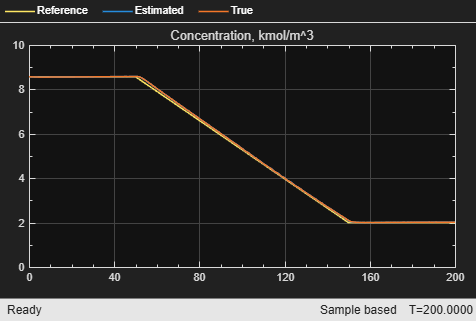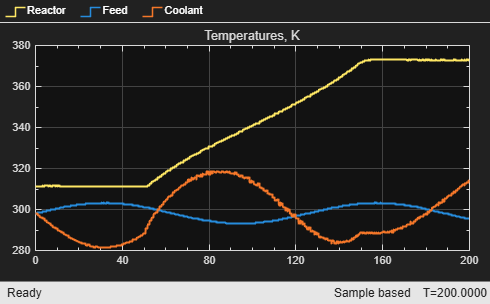The tracking and regulating performance is satisfactory.

### References

 Seborg, D. E., T. F. Edgar, and D. A. Mellichamp, Process Dynamics and Control, 2nd Edition, Wiley, 2004.